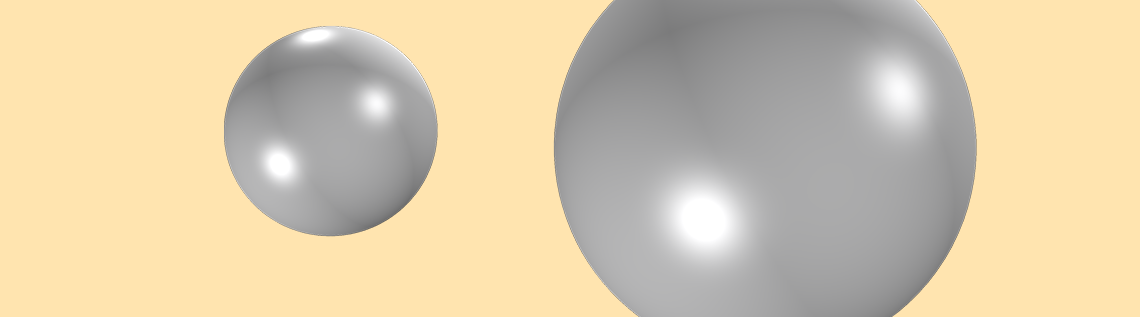# 如何在 COMSOL Multiphysics® 中计算电容矩阵

2017年 6月 7日

COMSOL Multiphysics® 软件中的电容计算很简单。如果你只有两个导体，最简单的方法是：将一个导体设为接地，另一个设为终端，然后求解。此时，内置变量会给出电容值。但是如果你有两个以上的导体，比如触摸屏、传输线和电容式传感器，怎么办呢？如果你对标准教科书术语感到困惑，请按照本博客中计算电容矩阵的工作示例进行操作。

### 什么是自电容？

Q=C\cdot V

C=4\pi\epsilon_0 R

### 互电容矩阵和麦克斯韦电容矩阵的比较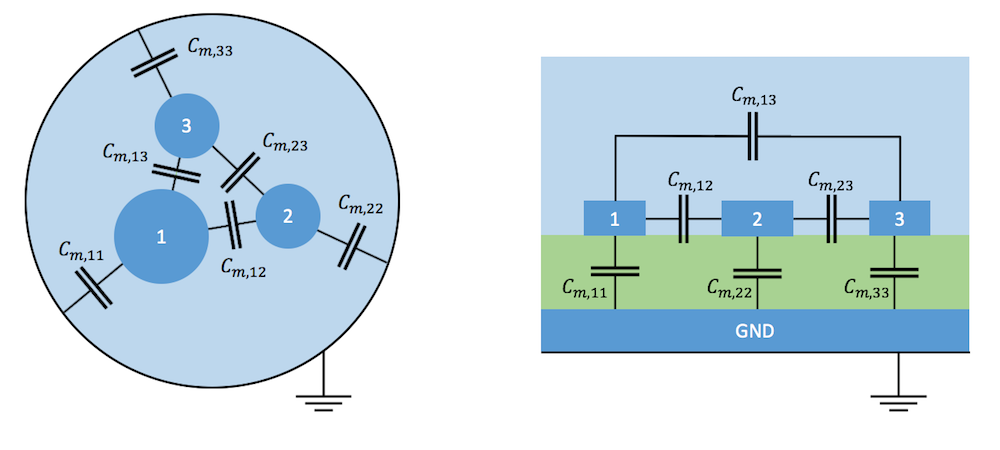带屏蔽层三芯电缆（左）和接地板上微带传输线之间互电容（右）的典型示例。从连续场模型到具有分立电容器的集总模型的转变意味着将导体收缩为点，同时将导体表面的电荷移动到它们之间的电容器的极板上。

\begin{bmatrix}C_{m,11} & C_{m,12} & \dots & C_{m,1N}\\
C_{m,21} & C_{m,22} & \dots & C_{m,2N} \\
\vdots & \vdots & \ddots & \vdots \\
C_{m,N1} & C_{m,N2} & \dots & C_{m,NN}\end{bmatrix}

\begin{pmatrix}Q_1 \\Q_2 \\\vdots \\Q_N\end{pmatrix} = \begin{bmatrix}C_{11} & C_{12} & \dots & C_{1N}\\
C_{21} & C_{22} & \dots & C_{2N} \\
\vdots & \vdots & \ddots & \vdots \\
C_{N1} & C_{N2} & \dots & C_{NN}\end{bmatrix}\begin{pmatrix}V_1 \\
V_2 \\\vdots \\V_N\end{pmatrix}

\begin{matrix}Q_1 &= &C_{m,11}V_1+C_{m,12}(V_1-V_2)+C_{m,13}(V_1-V_3)\\
&= & (C_{m,11}+C_{m,12}+C_{m,13})V_1-C_{m,12}V_2-C_{m,13}V_3)\end{matrix}

\begin{pmatrix}Q_1 \\
Q_2 \\\vdots \\Q_N\end{pmatrix} = \begin{bmatrix}\sum_{}{^N_{i=1}} C_{m,1i} & -C_{m,12} & \dots & -C_{m,1N}\\
-C_{m,21} & \sum_{}{^N_{i=1}} C_{m,2i} & \dots & -C_{m,2N}\\ \vdots & \vdots & \ddots & \vdots \\
-C_{m,N1} & -C_{m,N2} & \dots & \sum_{}{^N_{i=1}} C_{m,Ni}\end{bmatrix} \begin{pmatrix}V_1 \\
V_2 \\\vdots \\V_N\end{pmatrix}

### 操作示例：两个球体的互电容自麦克斯韦时代以来，这种系统的闭合表达式就已为人所知。我指的是 de Queiroz（2003）和 Lekner（2011）的两本现成出版物。三个麦克斯韦电容矩阵的表达式如下

C_{11}=Fab\sinh u \sum_{n=0}^{\infty} [a\sinh nu+ b\sinh (n+1)u]^{-1}
C_{22}=Fab\sinh u \sum_{n=0}^{\infty} [b\sinh nu+ a\sinh (n+1)u]^{-1}
C_{12}=-\frac{Fab}{c}\sinh u \sum_{n=1}^{\infty} [\sinh nu]^{-1}

\cosh u= \frac{c^2-a^2-b^2}
{2ab}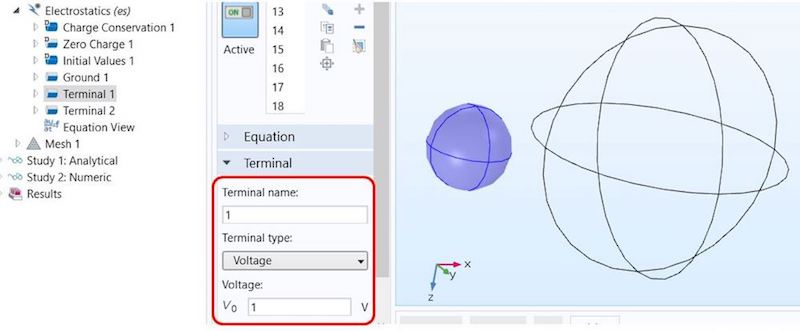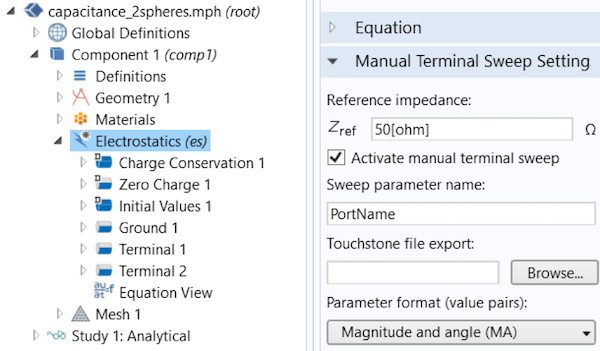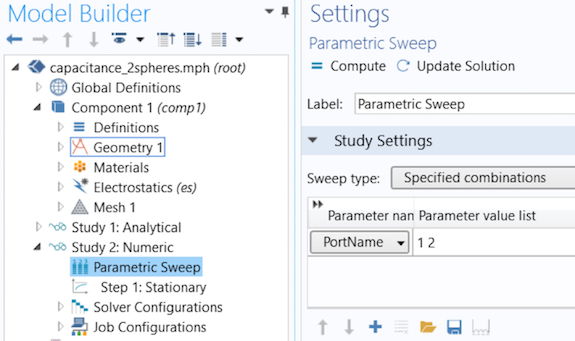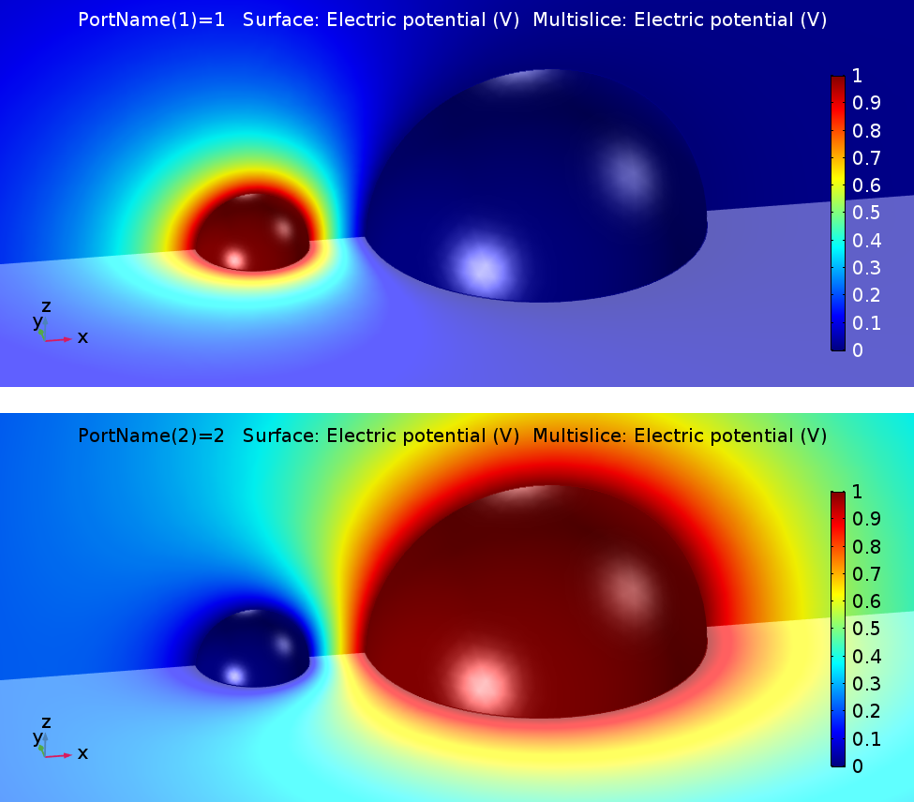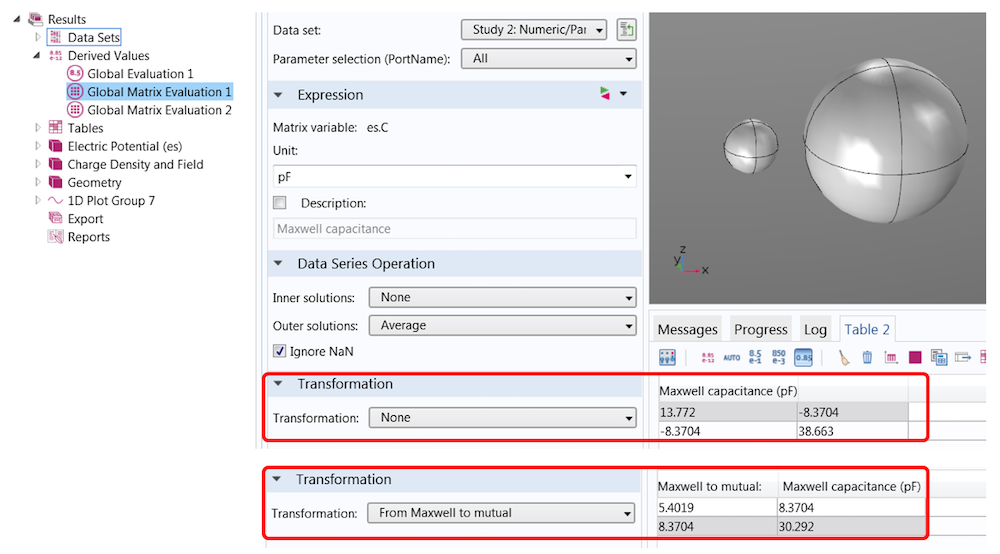\begin{bmatrix}C_{m,11} & C_{m,12}\\
C_{m,21} & C_{m,22}\end{bmatrix}=\begin{bmatrix}c_{11}+c_{12} & -c_{12}\\-c_{21} & c_{22}+c_{21}\end{bmatrix}

### 利用稳态源扫描和边界元法提高速度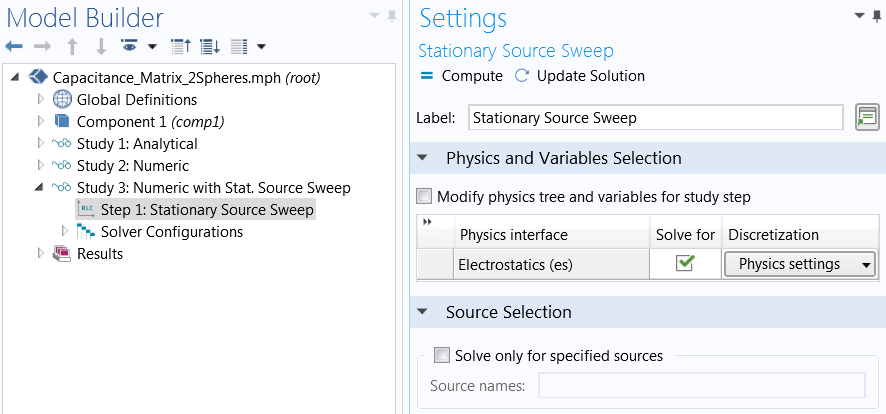COMSOL® 软件 5.3 版的另一个强大功能是静电中的边界元法（BEM）。与所有域（包括周围空气域）都需要网格的有限元法相比，边界元法避免了无限空间中的网格划分，从而减少了自由度的数量。你可以在使用 BEM 对电容式位置传感器建模教程中了解更多关于静电学中边界元和电容矩阵计算相结合的信息。

### 其他资源

• 浏览有关电容和边界元的博客文章：

### 参考文献

1. de Queiroz, A.C.M., 2003, “Capacitance Calculations“.
2. Lekner, J., 2011, “Capacitance coefficients of two spheres”, Journal of Electrostatics 69(1):11-14.

#### 评论 (3)

##### 留言##### 锋 张
2021-02-24

great!##### 登锋 祝
2021-12-25

thanks##### 哲 刘
2022-07-05

1.全局矩阵没有预表达式
2.偶尔可以的时候，提取出的麦克斯韦电容矩阵不含C12